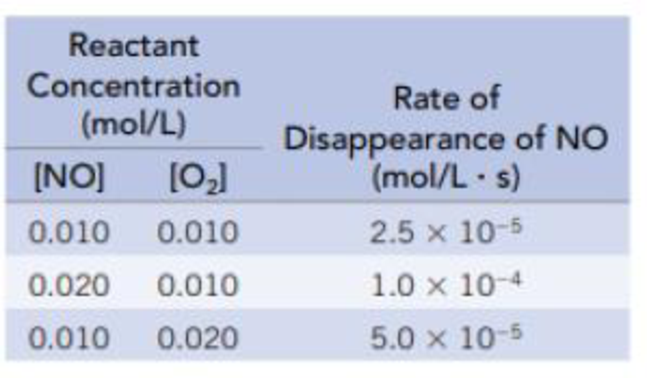# The data in the table are for the reaction of NO and O 2 at 660 K. NO(g) + ½ O 2 (g) → NO 2 (g) (a) Determine the order of the reaction for each reactant. (b) Write the rate equation for the reaction. (c) Calculate the rate constant. (d) Calculate the rate (in mol/L · s) at the instant when [NO] = 0.015 mol/L and [O 2 ] = 0.0050 mol/L. (e) At the instant when NO is reacting at the rate 1.0 × 10 −4 mol/L · s, what is the rate at which O 2 is reacting and NO 2 is forming?### Chemistry & Chemical Reactivity

9th Edition
John C. Kotz + 3 others
Publisher: Cengage Learning
ISBN: 9781133949640

#### Solutions

Chapter
Section### Chemistry & Chemical Reactivity

9th Edition
John C. Kotz + 3 others
Publisher: Cengage Learning
ISBN: 9781133949640
Chapter 14, Problem 11PS
Textbook Problem
147 views

## The data in the table are for the reaction of NO and O2 at 660 K.NO(g) + ½ O2(g) → NO2(g)(a) Determine the order of the reaction for each reactant. (b) Write the rate equation for the reaction. (c) Calculate the rate constant. (d) Calculate the rate (in mol/L · s) at the instant when [NO] = 0.015 mol/L and [O2] = 0.0050 mol/L. (e) At the instant when NO is reacting at the rate 1.0 × 10−4 mol/L · s, what is the rate at which O2 is reacting and NO2 is forming?

(a)

Interpretation Introduction

Interpretation:

The order of the reaction for each reactant has to be determined

Concept Introduction:

Rate law or rate equation: The relationship between the reactant concentrations and reaction rate is expressed by an equation.

aA + bBxXRate of reaction = k [A]m[B]n

Order of a reaction: The order of a reaction with respect to a particular reactant is the exponent of its concentration term in the rate law expression, and the overall reaction order is the sum of the exponents on all concentration terms.

Rate constant, k: It is a proportionality constant that relates rate and concentration at a given temperature.

### Explanation of Solution

The reaction rate of the chemical reaction is given as,

Reaction Rate = k [NO]m[O2]n,where m, and n are orders of the reactants.Givenreaction: NO(g)+ 1/2 O2(g)NO2(g)Findorderofthereaction:_Comparingfirsttwoexperiments1and2,rate1=[NO]m[O2]n, rate1 = 2.5×10-5mol/L.srate2 = k [NO]m[O2]n, rate2 = 1.0×10-4mol/L.srate2rate1=k [NO]m[O2]nk [NO]m[O2]n1.0×10-4mol/L.s2.5×10-5mol/L.s=(0.020)m(0.010)n(0.010)m(0

(b)

Interpretation Introduction

Interpretation:

The rate equation for the reaction has to be written.

Concept Introduction:

Rate law or rate equation: The relationship between the reactant concentrations and reaction rate is expressed by an equation.

aA + bBxXRate of reaction = k [A]m[B]n

Order of a reaction: The order of a reaction with respect to a particular reactant is the exponent of its concentration term in the rate law expression, and the overall reaction order is the sum of the exponents on all concentration terms.

Rate constant, k: It is a proportionality constant that relates rate and concentration at a given temperature.

(c)

Interpretation Introduction

Interpretation:

The rate constant has to be calculated.

Concept Introduction:

Rate law or rate equation: The relationship between the reactant concentrations and reaction rate is expressed by an equation.

aA + bBxXRate of reaction = k [A]m[B]n

Order of a reaction: The order of a reaction with respect to a particular reactant is the exponent of its concentration term in the rate law expression, and the overall reaction order is the sum of the exponents on all concentration terms.

Rate constant, k: It is a proportionality constant that relates rate and concentration at a given temperature.

(d)

Interpretation Introduction

Interpretation:

The rate in (mol/L.s) has to be calculated.

Concept Introduction:

Rate law or rate equation: The relationship between the reactant concentrations and reaction rate is expressed by an equation.

aA + bBxXRate of reaction = k [A]m[B]n

Order of a reaction: The order of a reaction with respect to a particular reactant is the exponent of its concentration term in the rate law expression, and the overall reaction order is the sum of the exponents on all concentration terms.

Rate constant, k: It is a proportionality constant that relates rate and concentration at a given temperature.

(e)

Interpretation Introduction

Interpretation:

The rate at which O2 reacts and NO2 forms has to be given.

Concept Introduction:

Rate law or rate equation: The relationship between the reactant concentrations and reaction rate is expressed by an equation.

aA + bBxXRate of reaction = k [A]m[B]n

Order of a reaction: The order of a reaction with respect to a particular reactant is the exponent of its concentration term in the rate law expression, and the overall reaction order is the sum of the exponents on all concentration terms.

Rate constant, k: It is a proportionality constant that relates rate and concentration at a given temperature.

### Still sussing out bartleby?

Check out a sample textbook solution.

See a sample solution

#### The Solution to Your Study Problems

Bartleby provides explanations to thousands of textbook problems written by our experts, many with advanced degrees!

Get Started

Find more solutions based on key concepts
Name the following molecular compounds a ClF4 b CS2 c PF3 d SF6

General Chemistry - Standalone book (MindTap Course List)

Vitamin K can be made from exposure to sunlight. can be obtained from most milk products. can be made by digest...

Nutrition: Concepts and Controversies - Standalone book (MindTap Course List)

Describe a polysome in bacterial cells.

Biology (MindTap Course List)

The __________ circuit carries blood to and from lungs. a. systemic b. pulmonary

Biology: The Unity and Diversity of Life (MindTap Course List)

Why is the following situation impossible? The object of mass m = 4.00 kg in Figure P6.6 is attached to a verti...

Physics for Scientists and Engineers, Technology Update (No access codes included)